Exploratory data analysis of interval-valued symbolic data with matrix visualization

Chiun-How Kaoa,b,  Junji Nakanoc, Sheau-Hue Shiehd, Yin-Jing Tienb, Han-Ming WueChuan-Kai Yanga, Chun-houh Chenb

a. Computer Graphics & Multimedia Lab., NTUST

b. Institute of Statistical Science, Academia Sinica, Taipei, Taiwan

c. The Institute of Statistical Mathematics, Tokyo, Japan

d. Center for Teacher Education, National Taipei University, New Taipei City, Taiwan

e. Department of Mathematics, Tamkang University, New Taipei City, Taiwan

Abstract

### Symbolic data analysis (SDA) has gained popularity over the past few years because of its potential for handling data having a dependent and hierarchical nature. Amongst many methods for analyzing symbolic data, exploratory data analysis (EDA: Tukey, 1977) with graphical presentation is an important one. Recent developments of graphical and visualization tools for SDA include zoom star, closed shapes, and parallel-coordinateplots. Other studies project high dimensional symbolic data into lower dimensional spaces using symbolic data versions of principal component analysis, multidimensional scaling, and self-organizing maps. Most graphical and visualization approaches for exploring symbolic data structure inherit the advantages of their counterparts for conventional (non-symbolic) data, but also their disadvantages. Here we introduce matrix visualization (MV) for visualizing and clustering symbolic data using interval-valued symbolic data as an example; it is by far the most popular symbolic data type in the literature and the most commonly encountered one in practice. Many MV techniques for visualizing and clustering conventional data are converted to symbolic data, and several techniques are newly developed for symbolic data. Various examples of data with simple to complex structures are brought in to illustrate the proposed methods.

 Figures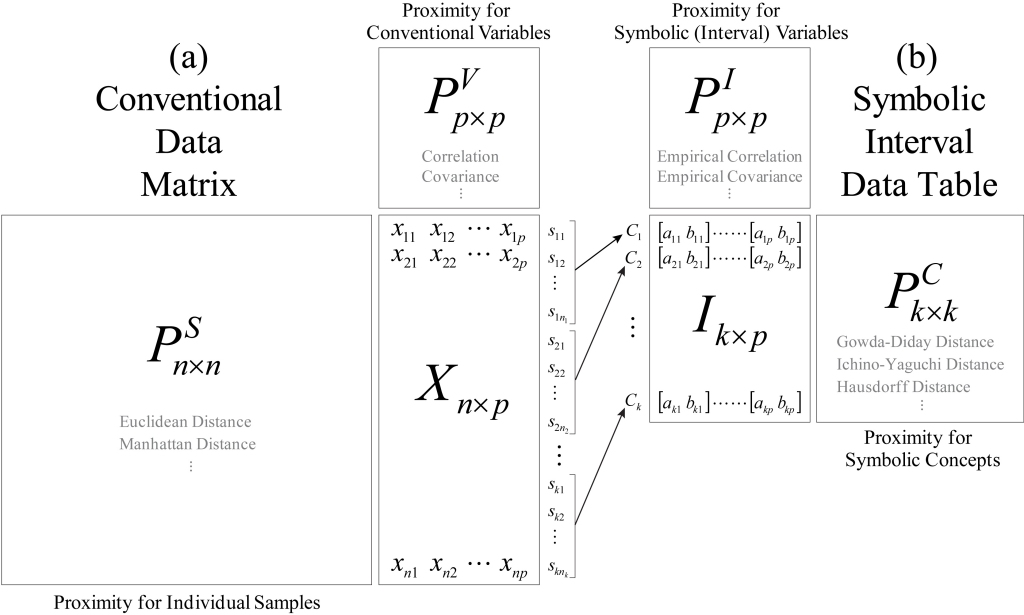### Figure 1: Diagram for related conventional data matrix and symbolic (interval type) data table with their corresponding proximity matrices for samples/concepts and variables.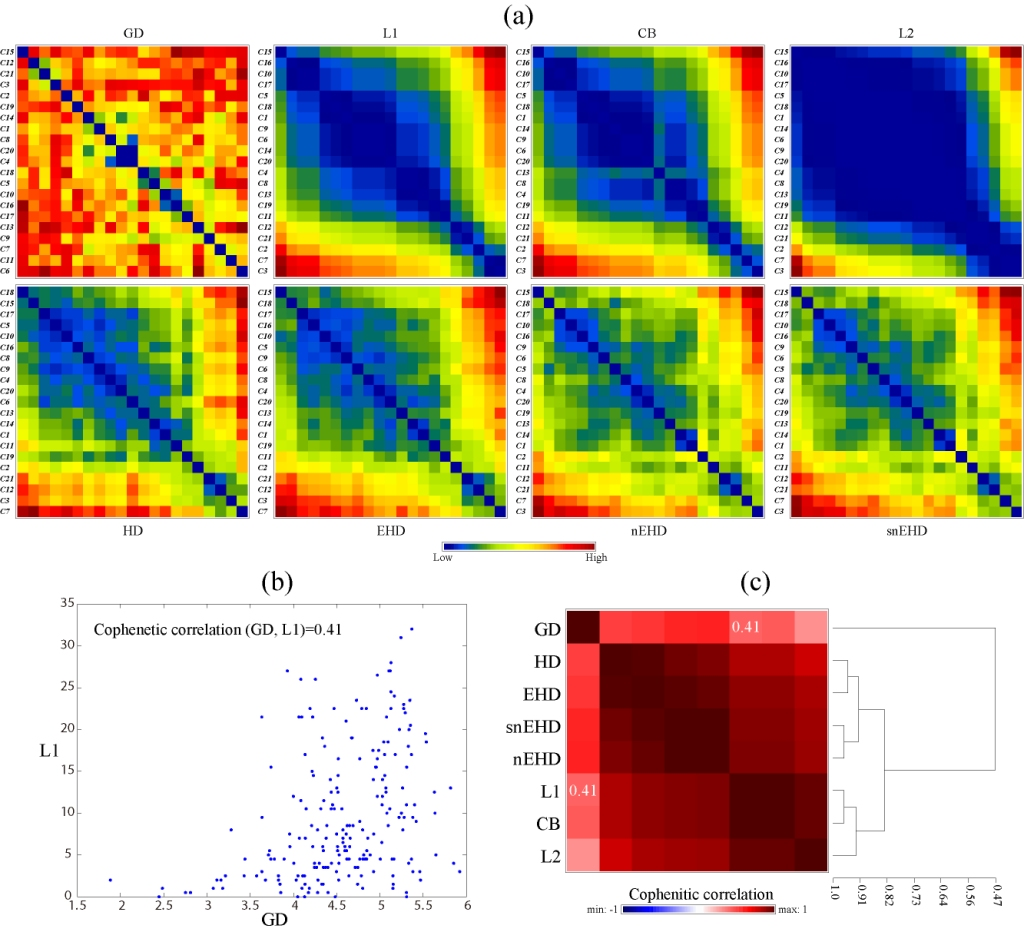### Figure 2: Comparison of between-concept distance measures. (a) Matrix visualization of 8 distance matrices each individually sorted by the HCT–R2E algorithm. (b) Cophenetic correlation of the Gowda–Diday distance (GD) matrix and the L1 distance matrix. (c) Matrix visualization and clustering of the pairwise cophenetic correlation among the 8 distance matrices. (For interpretation of the references to color in this figure legend, the reader is referred to the web version of this article.)### Figure 3: Color-coding scheme for interval-valued symbolic data using the Bats example. (a) Matrix condition. (b) Column condition. (c) Standardized data with different color maps.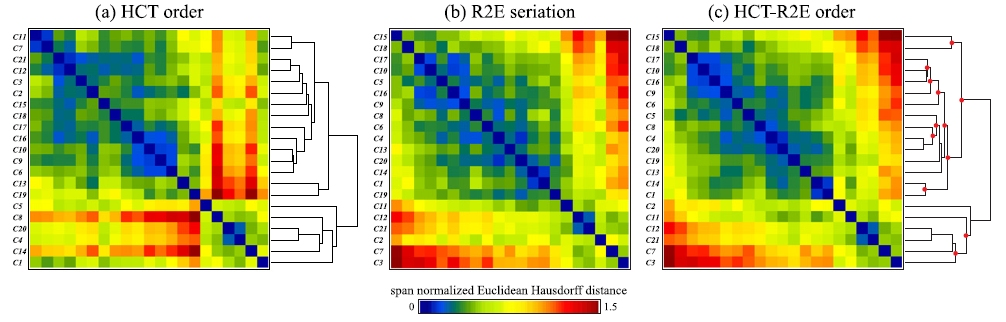### Figure 4: The HCT–R2E algorithm for the span normalized Euclidean Hausdorff distance matrix of the Bats data (Billard and Diday, 2006). (a) Matrix visualization with hierarchical clustering tree (HCT). (b) Matrix visualization with rank-two ellipse seriation (R2E). (c) Matrix visualization with R2E guided HCT (HCT–R2E); red dots on the dendrogram indicate intermediate nodes with flips. (For interpretation of the references to color in this figure legend, the reader is referred to the web version of this article.)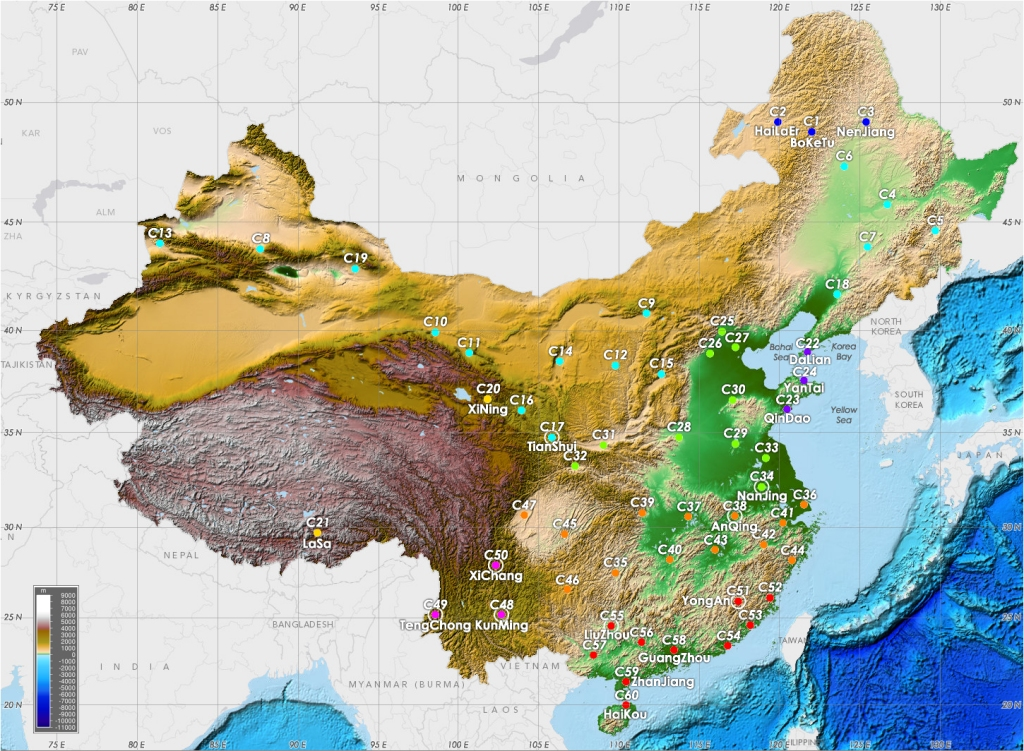### Figure 5: Sixty China meteorological stations in an elevation map. Colors for representing related clusters of stations identified from dendrogram structure in Fig. 6(a) are used to code each of the individual stations and white outer circle for those stations with number of disagreements ≥48. (For interpretation of the references to color in this figure legend, the reader is referred to the web version of this article.) Source: NOAA web page.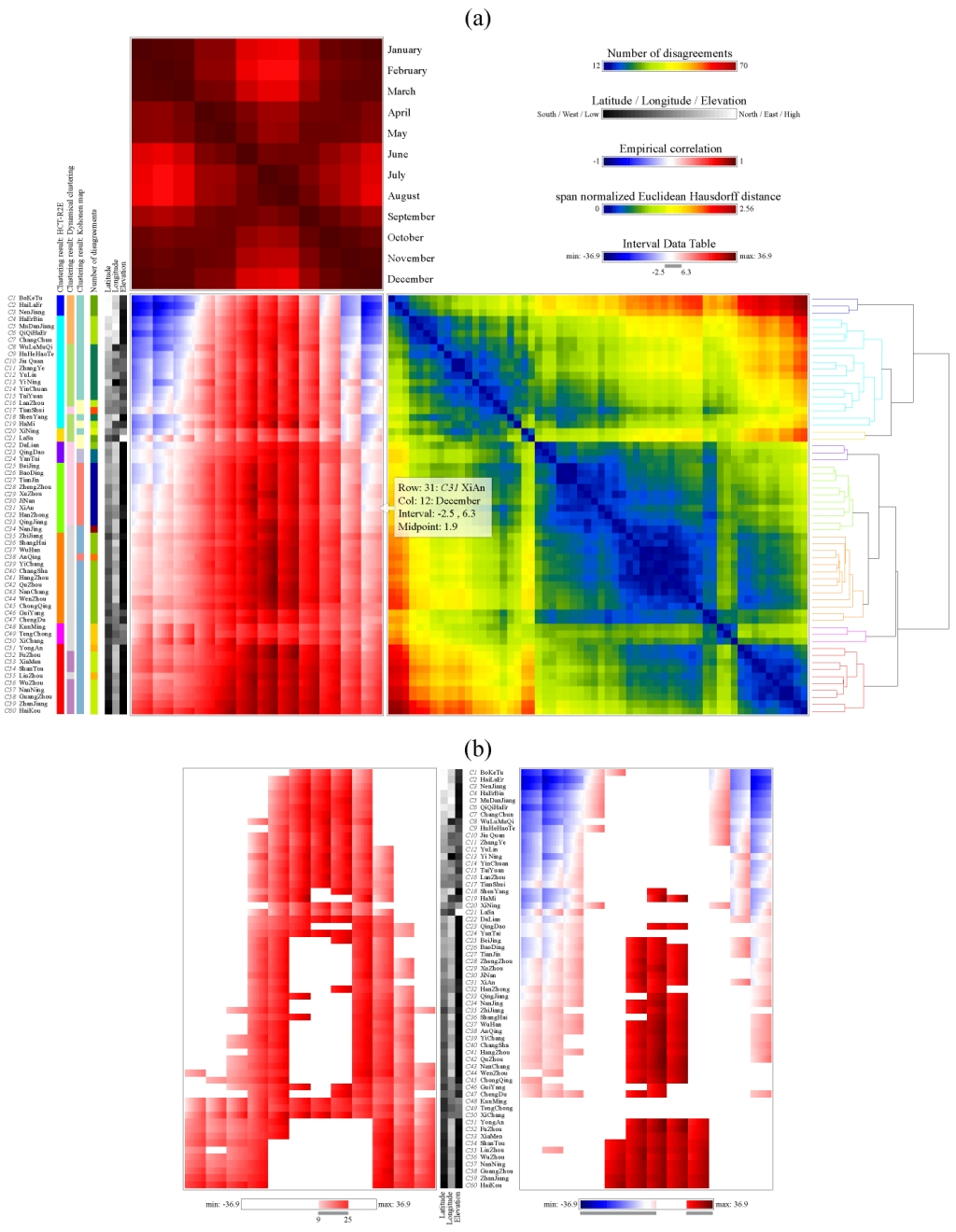### Figure 6: (a) Three MV maps sorted by HCT–R2E dendrograms for 12 monthly temperature range variables on 60 China meteorological stations data. (b) Midpoint condition range display for 60 China meteorological stations data in (a). Left panel: only temperature intervals with midpoint within the range of (9–25 °C) are displayed; right panel: only intervals with midpoint outside the range of (9–25 °C) are displayed.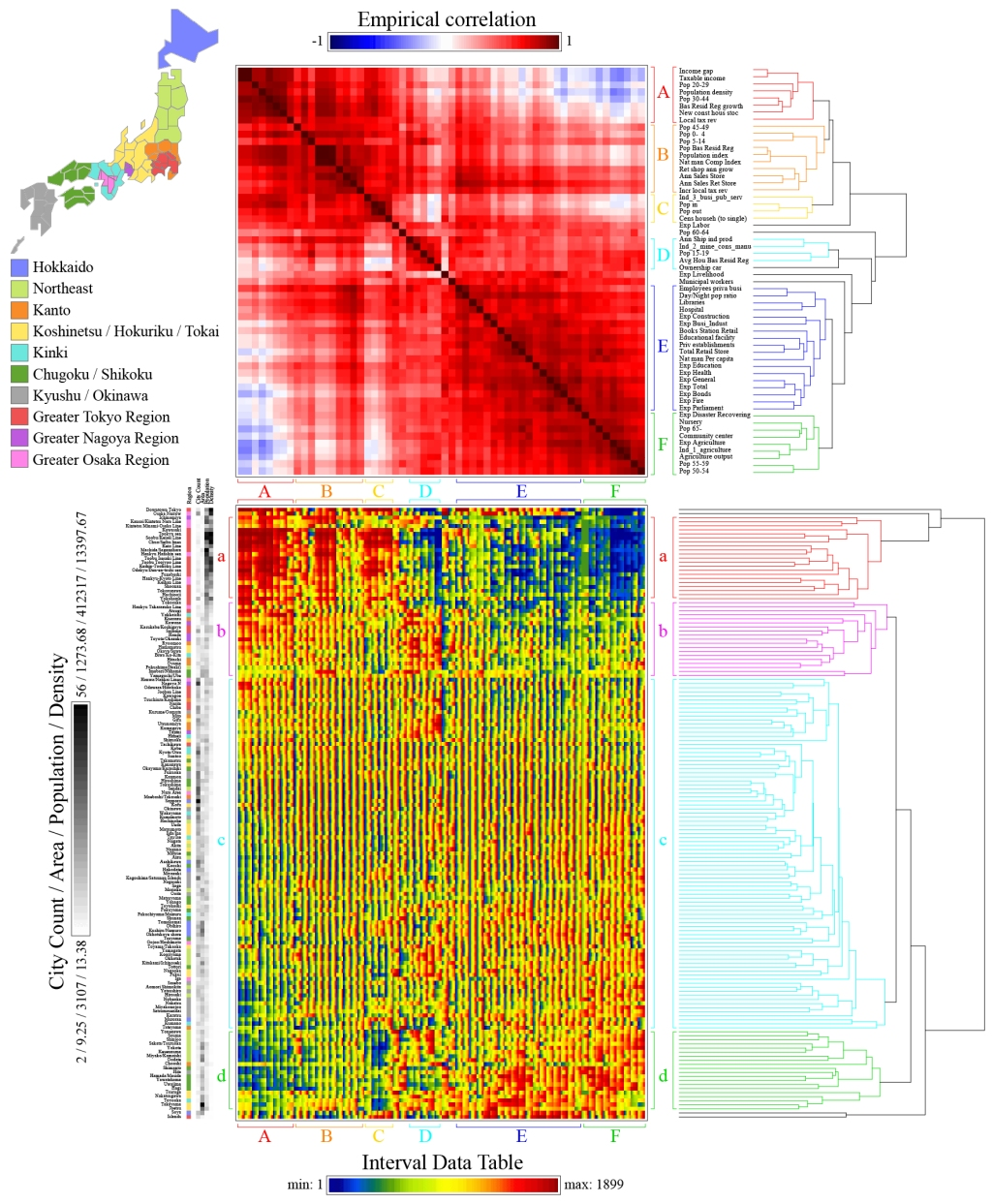### Figure 7: Two matrix maps for Minryoku 2010 data sorted also by HCT–R2E seriations: the 151 areas by 58 rank interval table, I151×58, the empirical correlation map for 58 manpower rank interval variables, PI58×58. The span normalized Euclidean Hausdorff distance map for 151 areas, PC151×151, is not shown due to limitation of space.